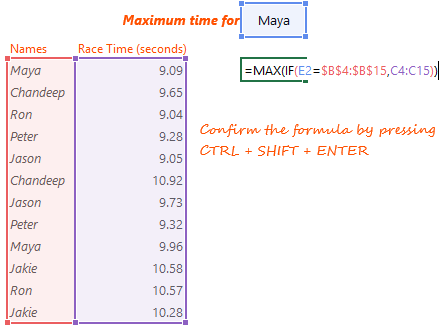##Often there are tasks in excel that seem impossible to perform. Array formulas can handle a lot of that impossibility and do miraculous things. The problem is that array formulas is one difficult topics in Excel

“With great formula power comes great complexity”

Chandeep’s lame claim to fame 😀

For that very fact I am going to discuss with you 5 extremely helpful array formulas that I find myself using very often.

• Cool fact 1 – You can just copy & paste the formula to make it work in your own scenario
• Not so cool fact 2 – Make sure you confirm the formula in the cell by pressing CTRL+SHIFT+ENTER. Array formulas don’t work without that

## #1 Counting Unique Values from a Range`=SUM(1/COUNTIF(\$B\$3:\$B\$14,\$B\$3:\$B\$14))`

This formula helps you to count of unique values in a range. Note a few things

1. \$B\$3:\$B\$14 is the range which contains values (including duplicates)
2. In the range Chandeep (with C as Caps) and chandeep (with c as lowercase) are not unique but they are the same

## #2 Extracting Unique Values from a Range`=IFERROR(INDEX(\$B\$4:\$B\$15,MATCH(0,COUNTIF(\$D\$3:D3,\$B\$4:\$B\$15),0)),'-')`

What if you wanted to not just count but also extract the unique values from a range. The above formula can help you do that. Note a few things

1. \$B\$4:\$B\$15 is the range which contains (duplicate) values
2. \$D\$3:D3 is one cell above where you are writing this formula and the first part of that range is locked (\$D\$3)
3. The upper or lower case of the value does not make a difference here

## #3 Vlookup for Multiple Records`=IFERROR(INDEX(B\$4:B\$15,SMALL(IF(\$E\$2=\$B\$4:\$B\$15,ROW(\$B\$4:\$B\$15)-ROW(D\$3),''),ROWS(D\$4:D4))),'-')`

Use this formula if your range has duplicates and you want to extract all the records that match the value that you are looking for. A few things to note

1. B\$4:B\$15 is the range from which you want to extract multiple records. Note the rows are locked
2. \$E\$2 is the cell which you are looking for
3. D\$3 is one row above from where you start writing this formula
4. D\$4:D4 is an expanding range from the cell where you start writing the formula

## Finding Min Value IF the Condition is met`=MIN(IF(E2=B4:B15,C4:C15))`

Excel provides for a few conditional functions but not for – Find the minimum if a condition is met

1. B4:B15 is the range where you check for the condition
2. E2 contains your condition/criteria
3. C4:C15 contains the values from which you want to find the minimum for the mentioned criteria

## Finding Max Value IF the Condition is met`=MAX(IF(E2=B4:B15,C4:C15))`

This is exactly same as the MINIF, the only difference being that the formula used is =MAX

## 2 Quick Action Items

1. If you have an array formula that you use very often, please share it with all Goodly people in the comments
2. In the comments, mention which formula from above do you think will be super helpful in your work?